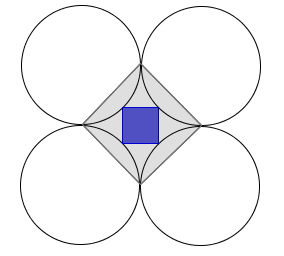Geometry Level 2With four tangent unit circles, we can form two squares. The large gray square is formed by the tangency points between the circles. The small blue one is formed by joining the midpoints of each arc that are formed by tangency points.

Find the difference of between the area of the $\color{#BBBBBB}{\text{gray square}}$ and that of the $\color{#3D99F6} {\text{blue square}}$ to 2 decimal places.

×